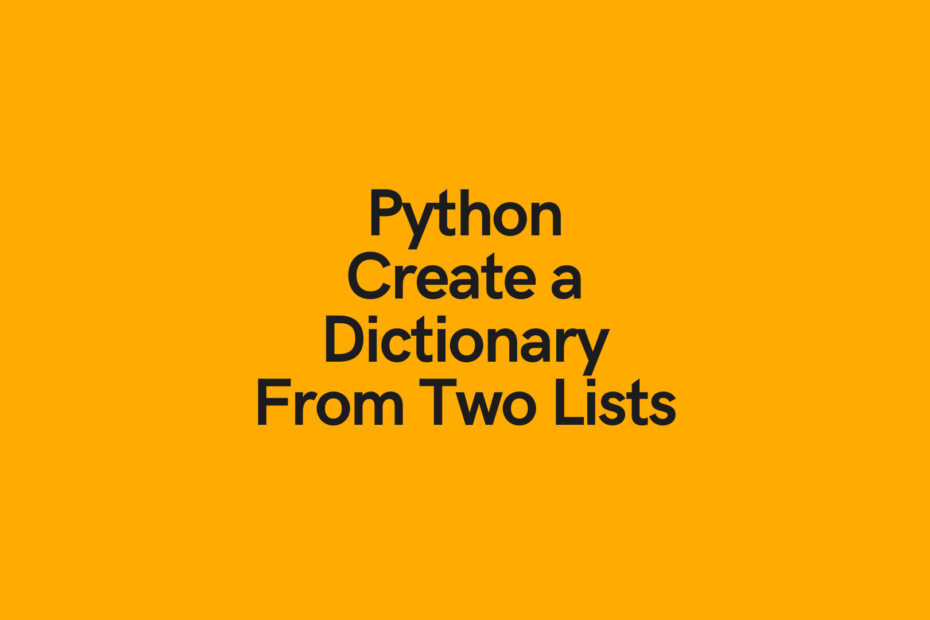# Python: Create Dictionary From Two ListsIn this post, you’ll learn how to use Python to create a dictionary from two lists. You’ll learn how to do this with the built-in `zip()` function, with a dictionary comprehension, and a naive for loop method. Finally, you’ll learn which of these methods is the best for highest performance.

``````names = ['nik', 'katie', 'james']
ages = [32, 31, 34]

dictionary = dict(zip(names, ages))
print(dictionary)

# Returns: {'nik': 32, 'katie': 31, 'james': 34}``````

## Covert Two Lists into a Dictionary with Python Zip

The Python `zip()` function is an incredibly helpful function to make iterating or multiple iterators much easier. To learn about the `zip()` function a bit more, check out my in-depth tutorial on zipping multiple Python lists.

Let’s see how we can use the `zip()` function to convert two Python lists into a dictionary:

``````names = ['nik', 'katie', 'james']
ages = [32, 31, 34]

dictionary = dict(zip(names, ages))
print(dictionary)

# Returns: {'nik': 32, 'katie': 31, 'james': 34}``````

Let’s see what we’ve done here:

1. We declared two lists, `names` and `ages`, which contains people’s names and their ages
2. We use the `zip()` function to turn this into a zip object containing tuples of the corresponding names and ages
3. Finally, we use the `dict()` function to convert the zip object into a dictionary, where the tuples are mapped as (key, value)

In the following section, you’ll learn how to use a dictionary comprehension to convert two Python lists into a dictionary.

## Covert Two Lists into a Dictionary with a Dictionary Comprehension

Dictionary comprehensions are a fun way to loop over items and create a resulting dictionary. To learn more about dictionary comprehensions, check out my in-depth tutorial here.

Let’s work with the two lists that we worked with above and use a Python dictionary comprehension to create a dictionary:

``````names = ['nik', 'katie', 'james']
ages = [32, 31, 34]

dictionary = {names[i]:ages[i] for i in range(len(names))}
print(dictionary)

# Returns: {'nik': 32, 'katie': 31, 'james': 34}``````

The way that this dictionary comprehension works is as below:

1. We loop over the range(len(names)), meaning that we loop over the numbers 0 through 2.
2. We assign the dictionary key the index item of the `names` list
3. We assign the dictionary value the index item of the `ages` list

Dictionary comprehensions can be a bit tricky to get a hang of, but they are fun to write!

## Covert Two Lists into a Dictionary with a For Loop

Wherever you can use a comprehension, you can also use a for-loop. Let’s see how we can loop over two lists to create a Python dictionary out of the two lists.

We will want to loop over the `range()` of the length of one of the lists and access the items.

Let’s give this a try and see how we can build on it:

``````names = ['nik', 'katie', 'james']
ages = [32, 31, 34]

dictionary = dict()

for item in range(len(names)):
key = names[item]
value = ages[item]
dictionary[key] = value

print(dictionary)

# Returns: {'nik': 32, 'katie': 31, 'james': 34}``````

Let’s explore what we’ve done here:

1. We create an empty dictionary using the `dict()` function
2. We loop over each in the `range()` function
3. We access the value of the `names` index and assign it to the variable `key`
4. We do the same for the `ages` list and assign it to `value`
5. We assign the dictionary key and value using direct assignment

We can also simplify this quite a bit by simply not assigning the variables first:

``````names = ['nik', 'katie', 'james']
ages = [32, 31, 34]

dictionary = dict()

for item in range(len(names)):
dictionary[names[item]] = ages[item]

print(dictionary)
# Returns: {'nik': 32, 'katie': 31, 'james': 34}``````

Now that you’ve learned how to combine two lists into a dictionary, let’s find out which of these methods is the one with the best performance!

## What is the Most Efficient Way to Covert Two Lists into a Dictionary?

Now that you have learned three different methods to turn two Python lists into a dictionary, let’s see which of these methods has the highest performance.

We can create a decorator to time these items and quickly turn them into functions:

``````import time

def time_it(func):
"""Print the runtime of a decorated function."""
def wrapper_time_it(*args, **kwargs):
start_time = time.perf_counter()
value = func(*args, **kwargs)
end_time = time.perf_counter()
run_time = end_time - start_time
print(f"Finished {func.__name__!r} in {run_time:.10f} seconds")
return value
return wrapper_time_it

@time_it
def zip_dict(list_a, list_b):
return dict(zip(list_a, list_b))

@time_it
def dict_comprehension(list_a, list_b):
return {list_a[i]:list_b[i] for i in range(len(list_a))}

@time_it
def for_loop(list_a, list_b):
dictionary = dict()

for item in range(len(list_a)):
dictionary[list_a[item]] = list_b[item]

return dictionary

list_a = range(10000000)
list_b = range(10000000)

zip_dict(list_a, list_b)
dict_comprehension(list_a, list_b)
for_loop(list_a, list_b)

# Returns:
# Finished 'zip_dict' in 0.8525682000 seconds
# Finished 'dict_comprehension' in 2.9896479000 seconds
# Finished 'for_loop' in 3.2348929000 seconds``````

From this, we can see that the `zip()` function method is significantly faster. It also happens to be more memory efficient since it only loads the data as it needs to!

## Conclusion

In this post, you learned three different ways two turn two Python lists into a dictionary. You learned how to accomplish this using the built-in `zip()` function, a dictionary comprehension, and a for loop. You also learned that the `zip()` function is the most efficient way of doing this, saving you nearly 3/4 of the time.

#### Nik Piepenbreier

Nik is the author of datagy.io and has over a decade of experience working with data analytics, data science, and Python. He specializes in teaching developers how to use Python for data science using hands-on tutorials.View Author posts

Tags:

## 2 thoughts on “Python: Create Dictionary From Two Lists”

1.This article saved my life, thank you so much to share it to us.
Best wishes. Happy new year!

1.Thank you so much! This made my day! Happy new year!